Transcendence of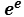The method here used to prove the transcendence of exp(e) is very similar to the one already used to demonstrate the transcendence of exp(algebraic number) (1).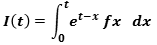is a holomorphic function, differentiable over the complex numbers (2) in every point of its domain, and its integrand is a polynomial of a certain degree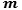, in which the exponent of the denominator slides all domain values [0,t]

Its input variables are conjugates indexed by a table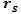made of r=rows and s=columns compatible with the modular form (3)

We exploit all the properties of complex conjugation including those about the odd-degree polynomials, in accordance with the complex conjugate root theorem.

We assume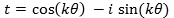, i.e. a conjugate in polar form, with k integer.
Each of the infinite values that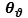can assume, is closely related to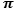or its multiple or its fraction. For this reason we must assume that the value of the multiple or the value of the fraction ofcan also be non-algebraic.
Then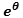returns algebraic values, (4)

Integrating by parts and assuming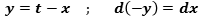, we get: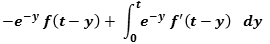then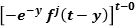then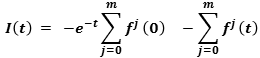with= degree of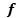and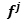= j-th derivative of f .

Let a symmetric polynomial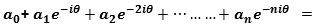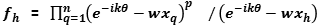with degree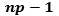, with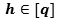,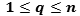,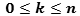, and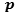is a Prime sufficiently large.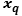are distinct algebraic complex conjugate linearly independent. Appropriate coefficients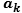and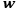makeroot of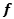This polynomial is never negative.

Then we use next polynomial with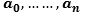integers non-zero , to verify the possibility of existence of an algebraic result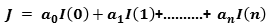=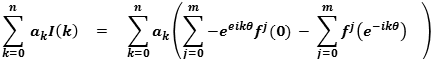=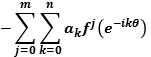(derivative of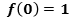= null).

Considering that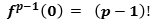by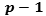derivations, and assuming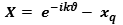, we can extract from, byderivations, the polynomial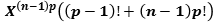, then , the minimal polynomial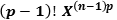is divisible by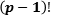and it follows that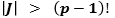Considering that a , n and t have not infinite values , so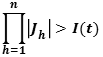is defined in a bounded set, therefore there must be a number greater than J . This number could be an arbitrary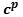. So we have :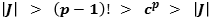(1)
let us avoid the immediate and trivial demonstration :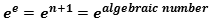(2)
The use of complex numbers ensures that every non-constant polynomial has a root, since the Fundamental Theorem of Algebra states that every non-constant polynomial with coefficients in C , has zeros in C , false in R (typical instance: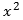has zero in complex numbers only).

(3)
a redundance of complex numbers in upper half-plane in which each point of each of the two axes is intersected by a two-dimensional table composed of complex numbers, id est an object in four spatial dimensions, which returns only positive values, not drawable on graph.

(4)
So, we get exp(algebraic number) again!